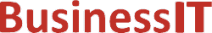O nás     Inzerce     KontaktSpolehlivé informace o IT již od roku 2011[Linux manuál]

# ldexp, ldexpf, ldexpl: načte exponent čísla s pohyblivou řádovou čárkou

Originální popis anglicky: ldexp, ldexpf, ldexpl - load exponent of a floating-point number

Návod, kniha: POSIX Programmer's Manual

## STRUČNĚ

#include <math.h>

double ldexp(double x, int exp);

float ldexpf(float x, int exp);

long double ldexpl(long double x, int exp );

## POPIS / INSTRUKCE

These functions shall compute the quantity x * 2**exp.
An application wishing to check for error situations should set errno to zero and call feclearexcept(FE_ALL_EXCEPT) before calling these functions. On return, if errno is non-zero or fetestexcept(FE_INVALID | FE_DIVBYZERO | FE_OVERFLOW | FE_UNDERFLOW) is non-zero, an error has occurred.

## NÁVRATOVÁ HODNOTA

Upon successful completion, these functions shall return x multiplied by 2, raised to the power exp.
If these functions would cause overflow, a range error shall occur and ldexp(), ldexpf(), and ldexpl() shall return ±HUGE_VAL, ±HUGE_VALF, and ±HUGE_VALL (according to the sign of x), respectively.
If the correct value would cause underflow, and is not representable, a range error may occur, and  either 0.0 (if supported), or  an implementation-defined value shall be returned.
If x is NaN, a NaN shall be returned.
If x is ±0 or ±Inf, x shall be returned.
If exp is 0, x shall be returned.
If the correct value would cause underflow, and is representable, a range error may occur and the correct value shall be returned.

## CHYBY / ERRORY

These functions shall fail if:
Range Error
The result overflows.
If the integer expression (math_errhandling & MATH_ERRNO) is non-zero, then errno shall be set to [ERANGE]. If the integer expression (math_errhandling & MATH_ERREXCEPT) is non-zero, then the overflow floating-point exception shall be raised.

These functions may fail if:
Range Error
The result underflows.
If the integer expression (math_errhandling & MATH_ERRNO) is non-zero, then errno shall be set to [ERANGE]. If the integer expression (math_errhandling & MATH_ERREXCEPT) is non-zero, then the underflow floating-point exception shall be raised.

The following sections are informative.

None.

## APPLICATION USAGE

On error, the expressions (math_errhandling & MATH_ERRNO) and (math_errhandling & MATH_ERREXCEPT) are independent of each other, but at least one of them must be non-zero.

None.

None.

## SOUVISEJÍCÍ

feclearexcept() , fetestexcept() , frexp() , isnan() , the Base Definitions volume of IEEE Std 1003.1-2001, Section 4.18, Treatment of Error Conditions for Mathematical Functions, <math.h> Portions of this text are reprinted and reproduced in electronic form from IEEE Std 1003.1, 2003 Edition, Standard for Information Technology -- Portable Operating System Interface (POSIX), The Open Group Base Specifications Issue 6, Copyright (C) 2001-2003 by the Institute of Electrical and Electronics Engineers, Inc and The Open Group. In the event of any discrepancy between this version and the original IEEE and The Open Group Standard, the original IEEE and The Open Group Standard is the referee document. The original Standard can be obtained online at http://www.opengroup.org/unix/online.html .
 2003 IEEE/The Open Group
©2011-2021 BusinessIT.cz, ISSN 1805-0522 | Názvy použité v textech mohou být ochrannými známkami příslušných vlastníků.
Provozovatel: Bispiral, s.r.o., kontakt: BusinessIT(at)Bispiral.com | Inzerce: Best Online Media, s.r.o., zuzana@online-media.cz
O vydavateli | Pravidla webu BusinessIT.cz a ochrana soukromí | Používáme účetní program Money S3 | pg(9263)